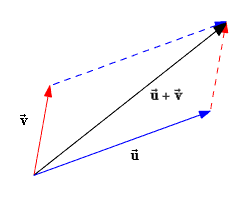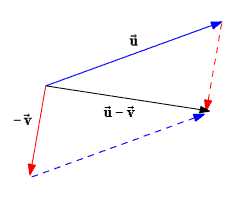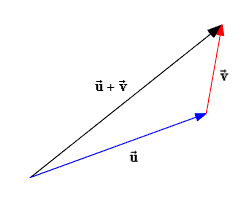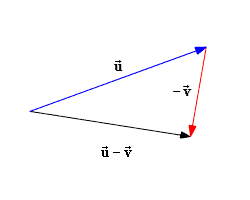To add or subtract two vectors, add or subtract the corresponding components.

Let $\stackrel{\to }{u}=⟨{u}_{1},{u}_{2}⟩$ and $\stackrel{\to }{v}=⟨{v}_{1},{v}_{2}⟩$ be two vectors.

Then, the sum of $\stackrel{\to }{u}$ and $\stackrel{\to }{v}$ is the vector

$\stackrel{\to }{u}+\stackrel{\to }{v}=⟨{u}_{1}+{v}_{1},{u}_{2}+{v}_{2}⟩$

The difference of $\stackrel{\to }{u}$ and $\stackrel{\to }{v}$ is

$\begin{array}{l}\stackrel{\to }{u}-\stackrel{\to }{v}=\stackrel{\to }{u}+\left(-\stackrel{\to }{v}\right)\\ \text{\hspace{0.17em}}\text{\hspace{0.17em}}\text{\hspace{0.17em}}\text{\hspace{0.17em}}\text{\hspace{0.17em}}\text{\hspace{0.17em}}\text{\hspace{0.17em}}\text{\hspace{0.17em}}\text{\hspace{0.17em}}\text{\hspace{0.17em}}\text{\hspace{0.17em}}=⟨{u}_{1}-{v}_{1},{u}_{2}-{v}_{2}⟩\end{array}$

The sum of two or more vectors is called the resultant. The resultant of two vectors can be found using either the parallelogram method or the triangle method .

## Parallelogram Method:

Draw the vectors so that their initial points coincide. Then draw lines to form a complete parallelogram. The diagonal from the initial point to the opposite vertex of the parallelogram is the resultant.1. Place both vectors $\stackrel{\to }{u}$ and $\stackrel{\to }{v}$ at the same initial point.

2. Complete the parallelogram. The resultant vector $\stackrel{\to }{u}+\stackrel{\to }{v}$ is the diagonal of the parallelogram.Vector Subtraction:

1. Complete the parallelogram.

2. Draw the diagonals of the parallelogram from the initial point.

## Triangle Method:

Draw the vectors one after another, placing the initial point of each successive vector at the terminal point of the previous vector. Then draw the resultant from the initial point of the first vector to the terminal point of the last vector. This method is also called the head-to-tail method .Vector Subtraction:Example:

Find (a) $\stackrel{\to }{u}+\stackrel{\to }{v}$ and (b) $\stackrel{\to }{u}-\stackrel{\to }{v}$ if $\stackrel{\to }{u}=⟨3,4⟩$ and $\stackrel{\to }{v}=⟨5,-1⟩$ .

Substitute the given values of ${u}_{1}$ , ${u}_{2}$ , ${v}_{1}$ and ${v}_{2}$ into the definition of vector addition.

$\begin{array}{l}\stackrel{\to }{u}+\stackrel{\to }{v}=⟨{u}_{1}+{v}_{1},{u}_{2}+{v}_{2}⟩\\ \text{\hspace{0.17em}}\text{\hspace{0.17em}}\text{\hspace{0.17em}}\text{\hspace{0.17em}}\text{\hspace{0.17em}}\text{\hspace{0.17em}}\text{\hspace{0.17em}}\text{\hspace{0.17em}}\text{\hspace{0.17em}}\text{\hspace{0.17em}}\text{\hspace{0.17em}}=⟨3+5,4+\left(-1\right)⟩\\ \text{\hspace{0.17em}}\text{\hspace{0.17em}}\text{\hspace{0.17em}}\text{\hspace{0.17em}}\text{\hspace{0.17em}}\text{\hspace{0.17em}}\text{\hspace{0.17em}}\text{\hspace{0.17em}}\text{\hspace{0.17em}}\text{\hspace{0.17em}}\text{\hspace{0.17em}}=⟨8,3⟩\end{array}$

Rewrite the difference $\stackrel{\to }{u}-\stackrel{\to }{v}$ as a sum $\stackrel{\to }{u}+\left(-\stackrel{\to }{v}\right)$ . We will need to determine the components of $-\stackrel{\to }{v}$ .

Recall that $-\stackrel{\to }{v}$ is a scalar multiple of $-1$ times $v$ . From the definition of scalar multiplication, we have:

$\begin{array}{l}-\stackrel{\to }{v}=-1⟨{v}_{1},{v}_{2}⟩\\ \text{\hspace{0.17em}}\text{\hspace{0.17em}}\text{\hspace{0.17em}}\text{\hspace{0.17em}}\text{\hspace{0.17em}}\text{\hspace{0.17em}}\text{\hspace{0.17em}}=-1⟨5,-1⟩\\ \text{\hspace{0.17em}}\text{\hspace{0.17em}}\text{\hspace{0.17em}}\text{\hspace{0.17em}}\text{\hspace{0.17em}}\text{\hspace{0.17em}}\text{\hspace{0.17em}}=⟨-5,1⟩\end{array}$

Now add the components of $\stackrel{\to }{u}$ and $-\stackrel{\to }{v}$ .

$\begin{array}{l}\stackrel{\to }{u}+\left(-\stackrel{\to }{v}\right)=⟨3+\left(-5\right),4+1⟩\\ \text{\hspace{0.17em}}\text{\hspace{0.17em}}\text{\hspace{0.17em}}\text{\hspace{0.17em}}\text{\hspace{0.17em}}\text{\hspace{0.17em}}\text{\hspace{0.17em}}\text{\hspace{0.17em}}\text{\hspace{0.17em}}\text{\hspace{0.17em}}\text{\hspace{0.17em}}\text{\hspace{0.17em}}\text{\hspace{0.17em}}\text{\hspace{0.17em}}\text{\hspace{0.17em}}\text{\hspace{0.17em}}\text{\hspace{0.17em}}\text{\hspace{0.17em}}=⟨-2,5⟩\end{array}$